Home

The pentagon

TYPE A PENTAGON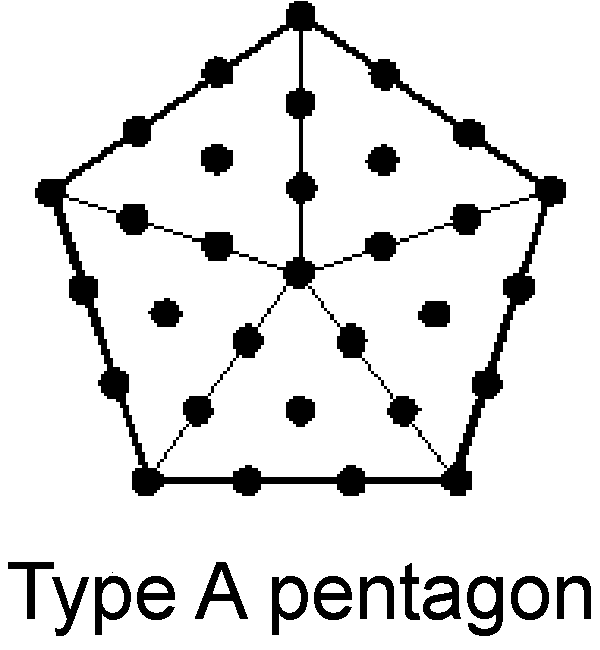(1) Number of yods = 31. The pentagon embodies the number of EL (אל), the Godname of Chesed. The central yod denotes the number value 1 of letter E (א, aleph) and the 30 yods surrounding the centre denote the number 30 of L (ל, lamed). 31 is the (16=42)th odd integer, the 11th prime number, the fifth Mersenne number and the third Mersenne prime defining the third perfect number 496, which is both the gematria number value of Malkuth and the 31st triangular number:

1 + 2 + 3 + ... + 31 = 496.

There are 15 yods on the boundary of the pentagon and 16 yods inside it, where 15 is the number value of YAH (YH), the older form of the Godname of Chokmah. The letter value 10 of Y (yod) denotes the 10 hexagonal yods on the sides of the pentagon and the letter value 5 of H (heh) denotes its five corners. 26 yods line the sides of its five sectors, where 26 is the number value of YAHWEH, the complete Godname assigned to Chokmah: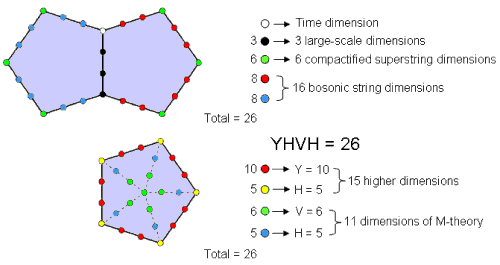26 yods line the sides of the two joined pentagons. They symbolise the 26 dimensions of space-time. The 4 yods in the root edge symbolise the 4 dimensions of Einstein's space-time continuum, the 6 corners outside the root edge denote the 6 compactified dimensions predicted by superstring theory and the 16 hexagonal yods on the 8 sides outside the root edge symbolise the 16 extra dimensions predicted by bosonic string theory. 26 yods line the sectors of the Type A pentagon. The letter values of YHVH, the Godname of Chokmah with number value 26, are the numbers of different types of yods.

This Godname also prescribes the two joined pentagons that are part of the inner Tree of Life because 26 yods line their sides.
(2) Sum of first 31 integers = 496. The pentagon arithmetically embodies the mysterious number at the heart of superstring theory, namely, the dimension 496 of the Yang-Mills gauge symmetry group describing interactions between 10-dimensional superstrings that are free of quantum anomalies (see also here). Sum of 16 (= 42) odd integers 1, 3, 5... 31 assigned to the internal yods of the pentagon = 256 = 44. Sum of 15 even integers 2, 4, 6... 30 assigned to the boundary yods = 240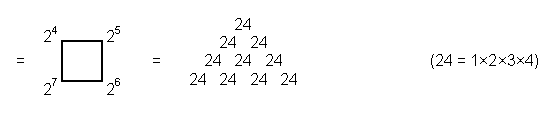(3) Sum of 30 (= 12 + 22 + 32 + 42) even integers on boundaries of a pair of pentagons = 240 + 240 = 480. The pentagons therefore encode the (240+240=480) non-zero roots of the superstring symmetry group E8×E8, which are symbolized in the inner Tree of Life as the (240+240=480) hexagonal yods of the (7+7) separate Type A polygons (see here).
(4) Sum of squares of first 31 integers = 10416 = 21×496. Sum of squares of 15 boundary integers = 4960

 496 496 496 = 496 496 496 496 496 496 496 ,

where 496 is the number value of Malkuth. It was pointed out in #5 at Occult Chemistry that 4960 is the number of space-time components of the 496 10-dimensional gauge fields mediating unified superstring interactions. This number is therefore encoded arithmetically in the pentagon as the sum of the squares of the 15 even integers on its boundary. Remarkably, 4960 is also the sum of the first 30 (= 12 + 22 + 32 + 42) triangular numbers which can be assigned to the 30 yods surrounding the centre of the pentagon or, alternatively, to the yods on the boundary of a pentagram constructed from 10 tetractyses with 61 yods, where 61 is the 31st odd integer:Sum of squares of 16 (= 42) internal integers = 5456where

 4 8 12 16 544 = 20 24 28 32 36 40 44 48 52 56 60 64 ,

The number 64 (=43), which is the centre of the 2nd-order tetractys shown above and the last of this 4×4 square array of integers 4 units apart, starting with 4, is the number of Nogah, the Mundane Chakra of Netzach, which is the fourth Sephirah of Construction. These representations show the basic connection between the Pythagorean Tetrad, tetractys and the arithmetic properties of the polygons.

(7) Two separate pentagons have 50 hexagonal yods. Enfolded, they have 48 hexagonal yods. The pentagon therefore embodies both the number of corners of the root edge + 7 separate Type A polygons and the latter by themselves.

(8) Number of yods on boundaries of two separate pentagons = 30 = 12 + 22 + 32 + 42. Enfolded, the number isThis is how the pentagon embodies the number of the Godnames EL, YAH & YAHWEH.

(9) Two separate pentagons have 62 yods made up of two centres, 40 yods on the sides of their 10 tetractyses, 10 corners and 10 centres of tetractyses. Each Tree in CTOL generates its own pair of seven polygons. In particular, the 10-tree prescribed by the Godname of Malkuth and denoting the 10 Sephiroth generates 10 pairs of such sets of polygons. The 20 pentagons contain 620 yods consisting of 20 centres, 400 yods on the boundaries of (50+50=100) tetractyses and 200 corners of pentagons or centres of tetractyses. These numbers are the letter values of Kether:

KTR = 620 = 20 + 400 + 200.

The pentagon can be said in this way to encode the numbers of the names of the first and last Sephiroth.

TYPE B PENTAGON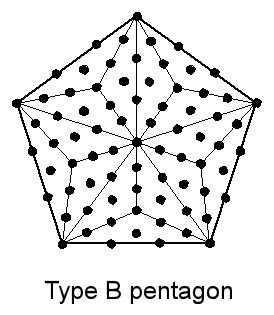(1) The pentagon has 76 yods. It embodies the number 76 of YAHWEH ELOHIM, the Godname of Tiphareth. Of these, 61 are internal yods and 65 are hexagonal yods, i.e., it embodies also the number 65 of ADONAI, the Godname of Malkuth. 65 is the sum of the first 10 integers after 1:

2
3   4
65 =      5   6   7
8  9  10  11.

This shows how the number 10, the Decad, determines the Godname number of the tenth Sephirah. The 65 hexagonal yods comprise 50 hexagonal yods that line 15 tetractyses and 15 hexagonal yods at the centres of the latter. Including their 11 corners, the 76 yods consist of 26 yods that line the five sectors of the pentagon and 50 yods inside them. The yod composition of the Type B pentagon embodies the number 26 of YAHWEH and the number 50 of ELOHIM. The latter prescribes its geometry because 10 corners, 25 sides & 15 triangles (i.e., 50 geometrical elements) surround its centre.

Two separate Type B pentagons havehexagonal yods. The number of yods in two joined pentagons is 148, which is the number of Netzach. The number outside the root edge is

 10 20 30 40 144 = 11 21 31 41 12 22 32 42 13 23 33 43  .

The number of hexagonal yods in two joined Type B pentagons outside the root edge is 126. This is the sum of the values of the 11 combinations of letters appearing in AHIH, the Godname of Kether:

A = 5, H = 5, I = 10.

 A + H + I = 16 AH + AI + HI + HH = 42 AHI + AHH + HHI = 47 AHIH = 21 Total = 126

126 is also the arithmetic mean of the first 26 triangular numbers, showing how the number of YAHWEH determines this number. The number of hexagonal yods in two enfolded Type B pentagons is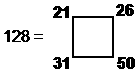i.e., 128 is the sum of the Godname numbers of the first four Sephiroth.

(2) The 76th odd integer is 151. This is the number of tree levels in the 49-tree . The Type B pentagon encodes a parameter of an important section of CTOL, namely, the cosmic physical plane that consists of the lowest 49 subplanes of consciousness.

(3) The sum of the first 76 integers is 2926. Assigning them to the yods of the pentagon, the sum of the 75 integers surrounding the central integer is 2925. We saw in the discussion of the square that this is the sum of the squares of the first 13 odd integers that can be assigned to the 13 internal yods of a Type A square (see here). The sum of the 61 internal integers = 1891 = 61×31.
(4) Sum of squares of first 76 integers = 149226 = 231×646, where 231 is the 21st triangular number and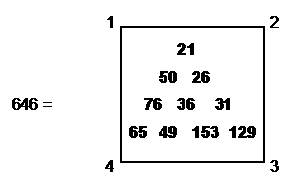Sum of squares of 61 internal integers = 77531 = 61×31×41, where 41 = 15 + 26.

TYPE A + TYPE B PENTAGONS
Two separate pentagons contain 107 yods (90 hexagonal yods). Two enfolded pentagons contain 103 yods, where 103 is the number of SABAOTH (“Hosts”) appearing in the Godnames of Netzach and Hod. They have 88 hexagonal yods, where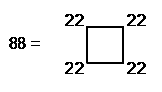and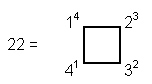The number 88 is a parameter of the inner Tree of Life, being the number of sides of the 47 sectors of the seven enfolded polygons (see here). It is also a parameter of the 3-dimensional Sri Yantra, being the number of points that create it (see Table 1 on page 2 of Article 35 and the diagram shown below). We discovered in the discussion of the triangle that two joined, Type B triangles have 88 yods: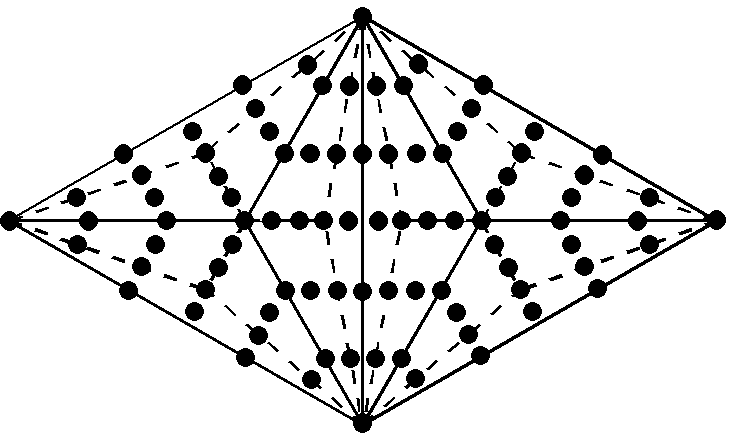It demonstrates that the pair of triangles is the seed source of the inner Tree of Life, its yods symbolizing the 88 straight lines that are needed to build the seven enfolded polygons that make up each half of it. Indeed, they also symbolize the central bindu and the 87 corners of the 43 triangles in the 3-dimensional Sri Yantra (see also here):(yods that are shown above composed of two half-circles with different colours denote corners of triangles in two adjacent layers of the Sri Yantra, one of which is directly above the other).

TYPE C PENTAGON
The Type C pentagon has 211 yods (2), that is, 206 yods other than its five defining corners. This is how the pentagon, whose diagonal width a and side b are in the proportion of the Golden Ratio: a/b = Φ = (1+√5)/2 = 1.618..., embodies the number of bones in the adult human skeleton. Many artists through the centuries have believed that this famous ratio determines the relative proportions of parts of the ideal human body. Whatever the truth of this claim, here is an indisputable, precise, mathematical connection between its bone composition and the Golden Proportion Φ. It is an illustration of the Tetrad Principle (see Article 1) governing holistic systems, whereby numbers that quantify them (in this case, the 206 bones in the human skeleton) are embodied in either the fourth member of a class of mathematical objects:

pentagon → Type A pentagon → Type B pentagon → Type C pentagon → etc,

or in the first four members of such a class.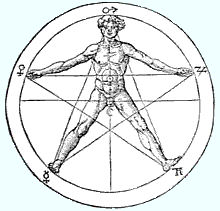The 16th-century philosopher Heinrich Agrippa drew a man over a pentagram inside a circle, implying a relationship to the Golden Ratio Φ. (From Heinrich Cornelius Agrippa's Libri tres de occulta philosophia).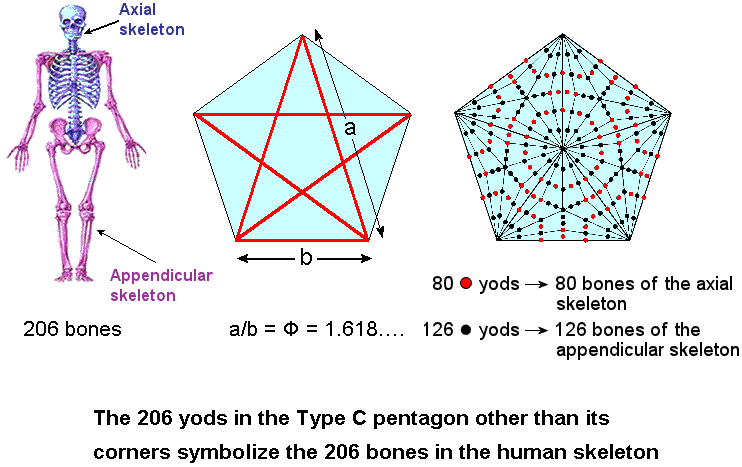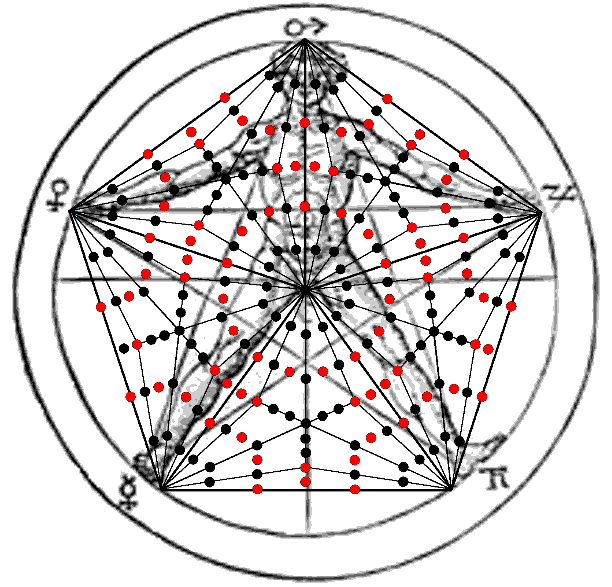The Type C pentagon has 26 corners & 70 sides of 45 triangles, i.e., 141 geometrical elements. 140 geometrical elements surround its centre, where 140 is the number of Masloth, the Mundane Chakra of Chokmah, whose Godname YAHWEH has the number 26. Two separate Type C pentagons have 282 geometrical elements, of which 50 are corners surrounding their centres. 282 is the number value of Aralim, the order of Angels assigned to Binah, the Sephirah opposite Chokmah in the Tree of Life whose Godname ELOHIM has the number value 50. Outside the root edge in each pentagon are 137 geometrical elements that surround its centre: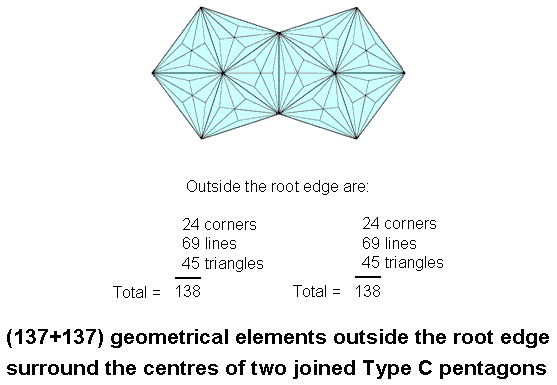The number 137 determining the fine-structure constant α = e2/ħc ≅ 1/137 well-known to physicists is embodied in the geometrical composition of the Type C pentagon, whilst the number of bones in the adult human skeleton is embodied in its yods other than its corners. Now, that's sacred geometry!

ELOHIM, the Godname of Binah, prescribes the pair of Type C pentagons because its number value 50 is the number of corners of their 90 triangles, whilst YAHWEH prescribes the 26 corners of the 45 triangles in each pentagon. As we saw earlier, YAHWEH prescribes the pair of pentagons because 26 yods line their sides.

The two joined Type C pentagons have 229 lines & triangles, where 229 is the 50th prime number. ELOHIM prescribes both the number of corners of the triangles in the two pentagons and the number of their sides & triangles!

The pair of joined Type C pentagons has 189 corners & sides, i.e., 187 corners & sides surround their two centres. 187 is the number of Auphanim, the Order of Angels assigned to Chokmah. 95 corners & sides in a Type C pentagon surround its centre, where 95 is the number value of Madim, the Mundane Chakra of Geburah.

A corner of each pentagon coincides with the centre of the decagon when it is part of the inner Tree of Life. This means that surrounding the centre of each pentagon are 136 geometrical elements outside the shared root edge that are intrinsic to this polygon in the sense that they do not belong to any other polygon in the inner Tree of Life. The pair of pentagons has 272 such geometrical elements, where 272 is the number value of Cherubim, the Order of Angels assigned to Yesod.

Notes
1. Tree levels are the horizontal divisions in overlapping Trees defined by Sephiroth that are shared between adjacent Trees (although not a Sephirah, Daath of a given Tree is counted as a tree level because it is Yesod of the next higher Tree). The n-tree has (3n+4) tree levels. The lowest Tree (n=1) has seven tree levels: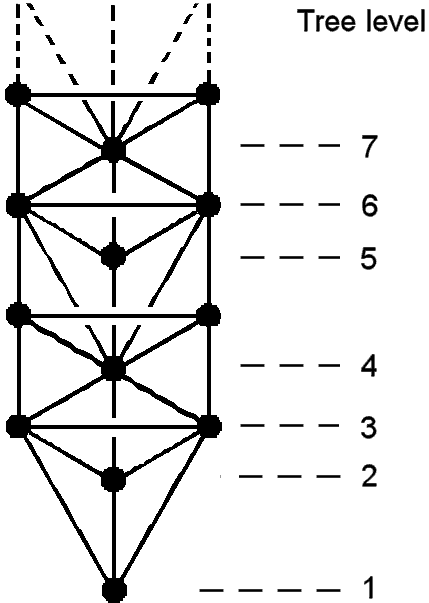The 49-tree has 151 tree levels. See also note 2 here.

2. Proof: The Type A n-gon has (6n+1) yods. Nine yods are added per sector in the Type B n-gon, so that it has (15n+1) yods. A further (9×3=27) yods are added per sector in the Type C n-gon, which has (42n+1) yods. The Type C pentagon (n=5) has 211 yods.

Home